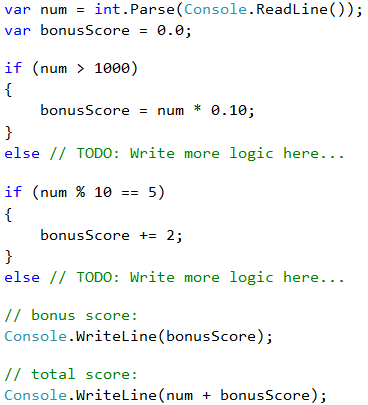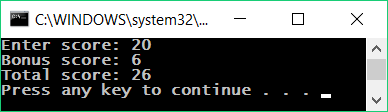Problem: Bonus Score

We have an integer – the number of points. Bonus score are charged on it, according to the rules described below. Write a program that calculates bonus score for this figure and total points with bonuses.

• If the number is up to 100 including, bonus score is 5.
• If the number is larger than 100, bonus score is 20% of the number.
• If the number is larger than 1000, bonus score is 10% of the number.
• Additional bonus score (accrued separately from the previous ones):
• for even number -> + 1 p.
• for number, that ends with 5 -> + 2 p.

Input Output
20 6
26
175 37
212
2703 270.3
2973.3
15875 1589.5
17464.5

Video: Bonus Score

Watch the video lesson about the solution of the "Bonus Score" problem: https://youtu.be/hstZ5rNJ7vs.

Hints and Guidelines

We can calculate the main and additional bonus score with a series of if-else-if-else statements. For the main bonus score we have 3 cases (when the entered number is up to 100, between 100 and 1000 and larger than 1000), and for extra bonus score – 2 more cases (when the number is even and odd).Here's an example of the program execution:Note that for this exercise, the judge is set to ignore anything that is not a number, so we can print not only the numbers, but also specifying text.